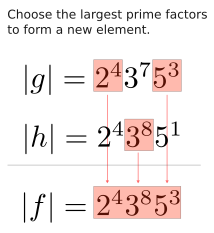Show Question
Math and science::Algebra::Aluffi

# Groups. All orders divides order of maximal element

### Order: divides order of maximal element

Let $$G$$ be a commutative group, and let $$g \in G$$ be an element with maximal finite order. Any other element with finite order has an order that divides $$|g|$$.

The same statement cannot be made for non-comutative groups.

Can you remember the proof?

#### Maximal finite order

Let $$g$$ be an element of group $$G$$. $$g$$ has maximal finite order iff all other elements with finite order have an order less than that of $$g$$.

### Proof essenceConsider elements $$g$$ and $$h$$ of group $$G$$, with orders as follows:

\begin{align} |g| &= 2^{4} 3^{7} 5^{3} \\ |h| &= 2^{4} 3^{8} 5^{1} \end{align}

$$|h|$$ doesn't divide $$|g|$$, as one prime is raised to a larger value. Commutativity allows us to create a new element $$f$$ that has an order whose prime factors are the maximum of those from $$|g|$$ and $$|h|$$. This order must be larger than $$|g|$$, so $$g$$ can't have maximal order.

In comparison, if $$k$$ is another element and $$|k|= 2^{4} 3^{5} 5^{1}$$, then this order divides $$|g|$$, as all primes are raised to a smaller power in $$|k|$$.

### Proof

Proof.

Let $$g$$ and $$h$$ be elements of a group $$G$$, with $$g$$ being an element of maximal finite order. Proceed by contradiction: assume that $$|h|$$ does not divide $$|g|$$.

We can express $$|g|$$ and $$|h|$$ in terms of their prime factorization:

\begin{align} |g| &= 2^{a}3^{b}5^{c}7^{d}... \\ |h| &= 2^{i}3^{j}5^{k}7^{l}... \end{align}

If every prime of $$|h|$$ is raised to a lower corresponding power than $$|g|$$, then $$|h|$$ would divide $$|g|$$. But by assumption it does not, so there must be at least 1 prime that is raised to a higher power in $$|h|$$ compared to $$|g|$$. Denote this prime by $$p$$. Focusing on this prime, we can now express $$|g|$$ and $$|h|$$ as so:

\begin{align} |g| &= p^{m}r \\ |h| &= p^{n}s \end{align}

Where $$r$$ and $$s$$ collect the other primes.

In a controlled way, we can create elements with smaller orders than $$|g|$$ and $$|h|$$. Consider first $$|g|$$. The element $$g^m$$ has an order that is $$m$$ times smaller than $$|g|$$, $$|g^m| = \frac{|g|}{m}$$. This is so, as $$m$$ is a factor of $$|g|$$. Complete this reduction by considering the element $$g^{m^p}$$, which is an element with order $$|g^{m^p}| = r$$. We have found an element whose order is $$|g|$$ but with the prime $$p$$ absent.

The same process can be repeated for $$|h|$$, and we now consider the element $$h^s$$, which has order $$|h^s| = p^n$$.

When we combine these two elements into a third element, the point of this process becomes clear. The element $$h^s g^{m^p}$$ has an order:

$|h^s g^{m^p} | = rp^n$

As $$n > m$$, we have found an element with order larger than that of $$|g|$$.

### Importance of commutativity

The proof above doesn't specifically mention where commutativity comes in. Can you spot it? Possibly surprisingly, implication requiring commutativity is the statement:

$|h^s| = p^n \land |g^{m^p}| = s \implies |h^s g^{m^p} | = rp^n$

Check Aluffi p49 (exercise 1.14) for an explation.

Aluffi p49